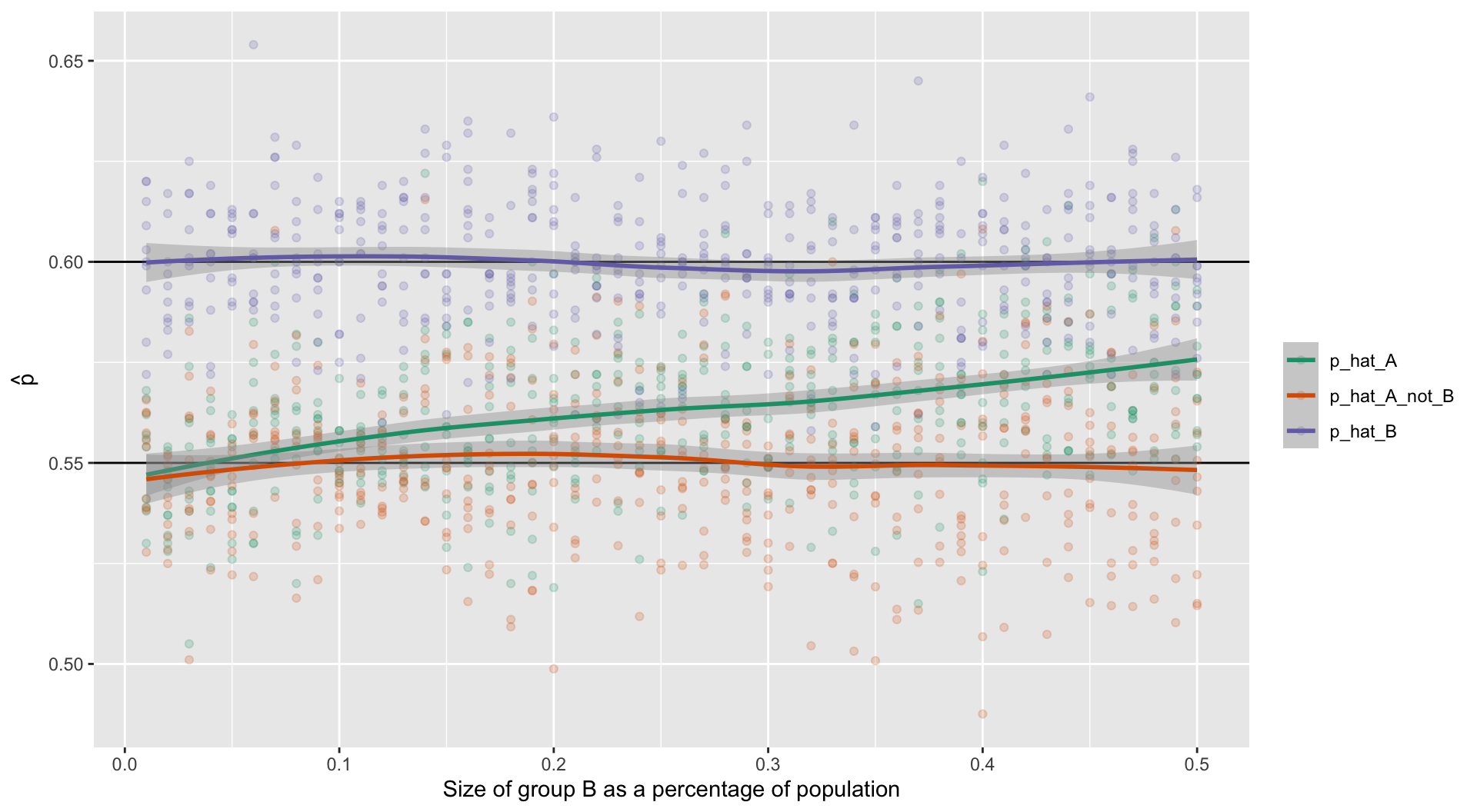March 18, 2020

## Meetup Presentations

• 6.23 & 6.25 Bonnie Cooper
• 6.27 Patrick Maloney
• 6.33 Manolis Manoli

## Independence Between Groups

Assume we have a population of 100,000 where groups A and B are independent with $$p_A = .55$$ and $$p_B = .6$$ and $$n_A = 99,000$$ (99% of the population) and $$n_B = 1,000$$ (1% of the population). We can sample from the population (that includes groups A and B) and from group B of sample sizes of 1,000 and 100, respectively. We can also calculate $$\hat{p}$$ for group A independent of B.

propA <- .55    # Proportion for group A
propB <- .6     # Proportion for group B
pop.n <- 100000 # Population size
sampleA.n <- 1000
sampleB.n <- 100

pop <- data.frame(
group = c(rep('A', pop.n * 0.99),
rep('B', pop.n * 0.01) ),
response = c(
sample(c(1,0), size = pop.n * 0.99, prob = c(propA, 1 - propA),
replace = TRUE),
sample(c(1,0), size = pop.n * 0.01, prob = c(propB, 1 - propB),
replace = TRUE) )
)

sampA <- pop[sample(nrow(pop), size = sampleA.n),]
sampB <- pop[sample(which(pop$group == 'B'), size = sampleB.n),] ## Independence Between Groups (cont.) $$\hat{p}$$ for the population sample mean(sampA$response)
##  0.561

$$\hat{p}$$ for the population sample, excluding group B

mean(sampA[sampA$group == 'A',]$response)
##  0.5606061

$$\hat{p}$$ for group B sample

mean(sampB\$response)
##  0.66

## Independence Between Groups (cont.)## High School & Beyond Survey

200 randomly selected students completed the reading and writing test of the High School and Beyond survey. The results appear to the right. Does there appear to be a difference?

data(hsb2) # in openintro package
geom_point(alpha=0.2, color='blue') + xlab('Test') + ylab('Score')